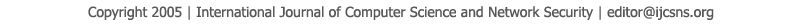To search, Click below search items.All Published Papers Search Service
 Title Author Year Keyword
 Title On the implementation of Implicit Methods to Solve Initial Values Problems for Ordinary Differential Equations Author Badreldin O. S. Elgabbani, Othman A. Alrusaini, Emad A. Shafie Citation Vol. 22  No. 3  pp. 517-524 Abstract The study presents a new method for solving initial value problems (IVPs) for ordinary differential equations (ODEs). The study successfully satisfied Butcher conditions and got a Jacobian matrix, The study successfully satisfied Butcher conditions and got a Jacobian matrix: a11, a12, a13, a21, λ ,a23, a31, a32, ? and then applying modified Newton, to find out the unknown vector ?i from iterative equation y_i=y_i+∂_i^ . The diagonal-implicit Range-Kutta, method submitted by this study solves the problem arising from application of an implicit multistage for linear or nonlinear algebra problems.The results achieved by using these parameters via Visual studio C++, compared to the ordinary differential equations that have an exact solution are more than impressive and get a shouted result, for example while comparing the exact solution of an ordinary differential equation with our numerical solution for the same problem, we get an approximation error 9.9873D-05. Therefore, with great confidence we can use this method with problems that need numerical solution. Keywords Ordinary differential equation; Butcher; Runge-Kutta; implicit;, Array URL http://paper.ijcsns.org/07_book/202203/20220366.pdf